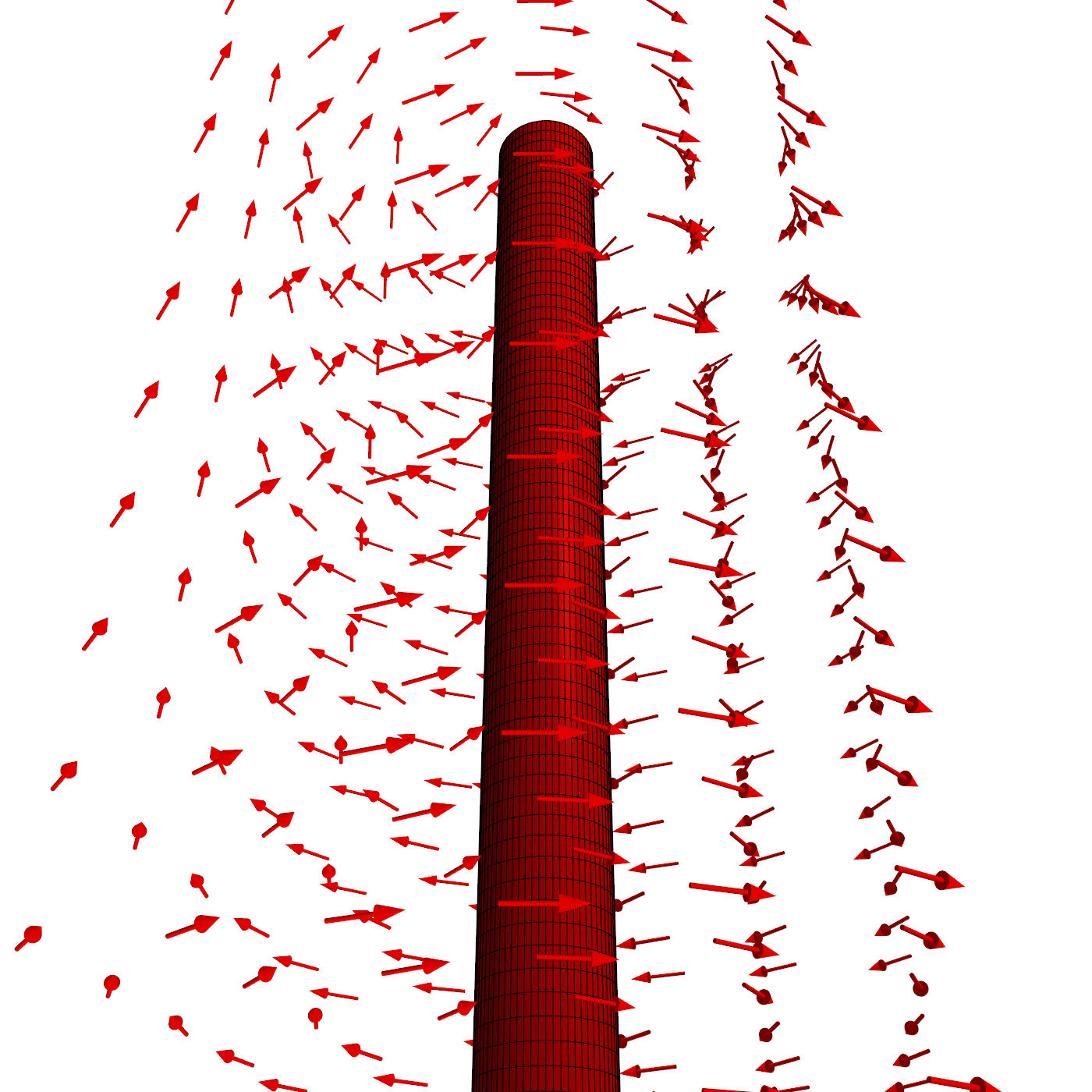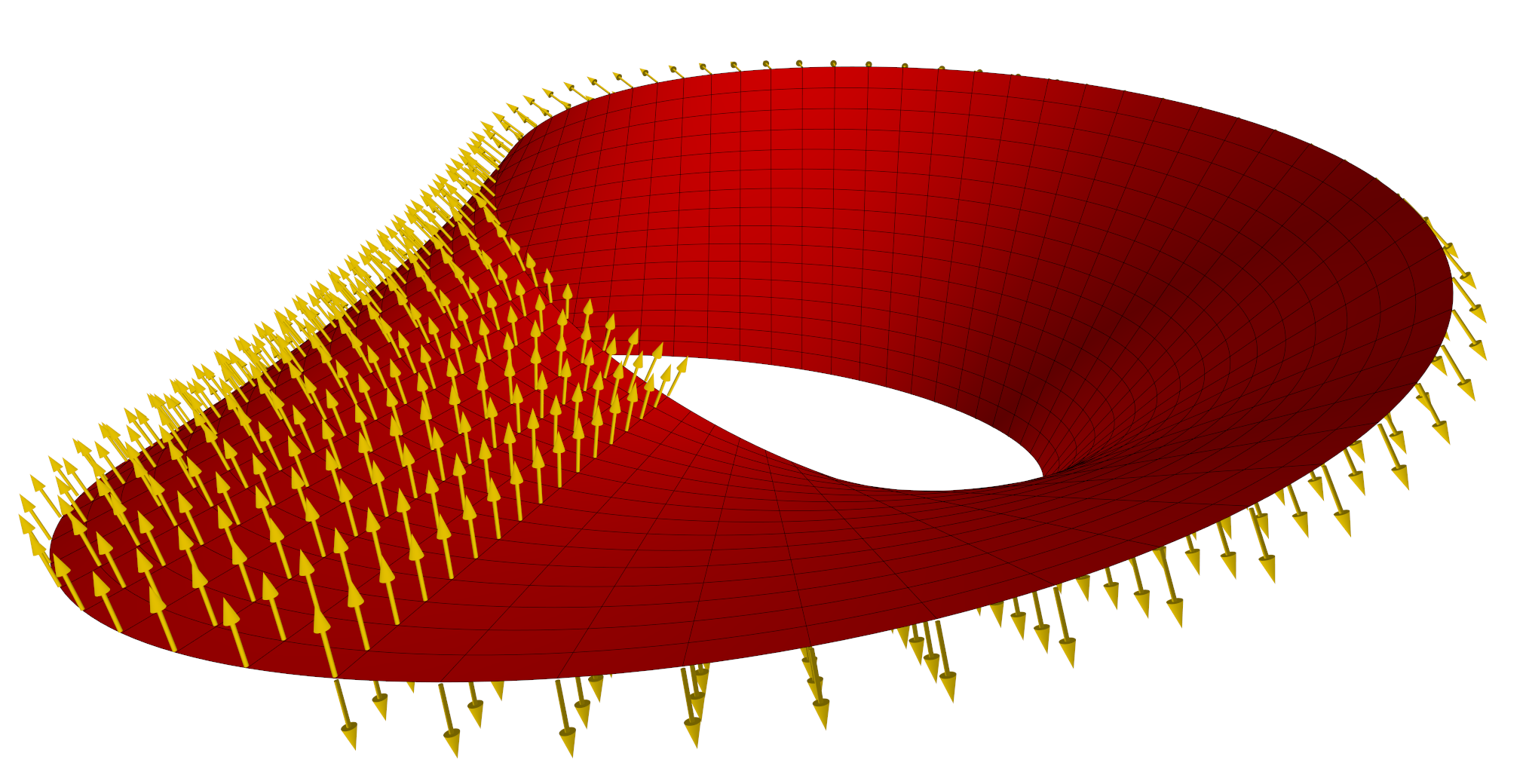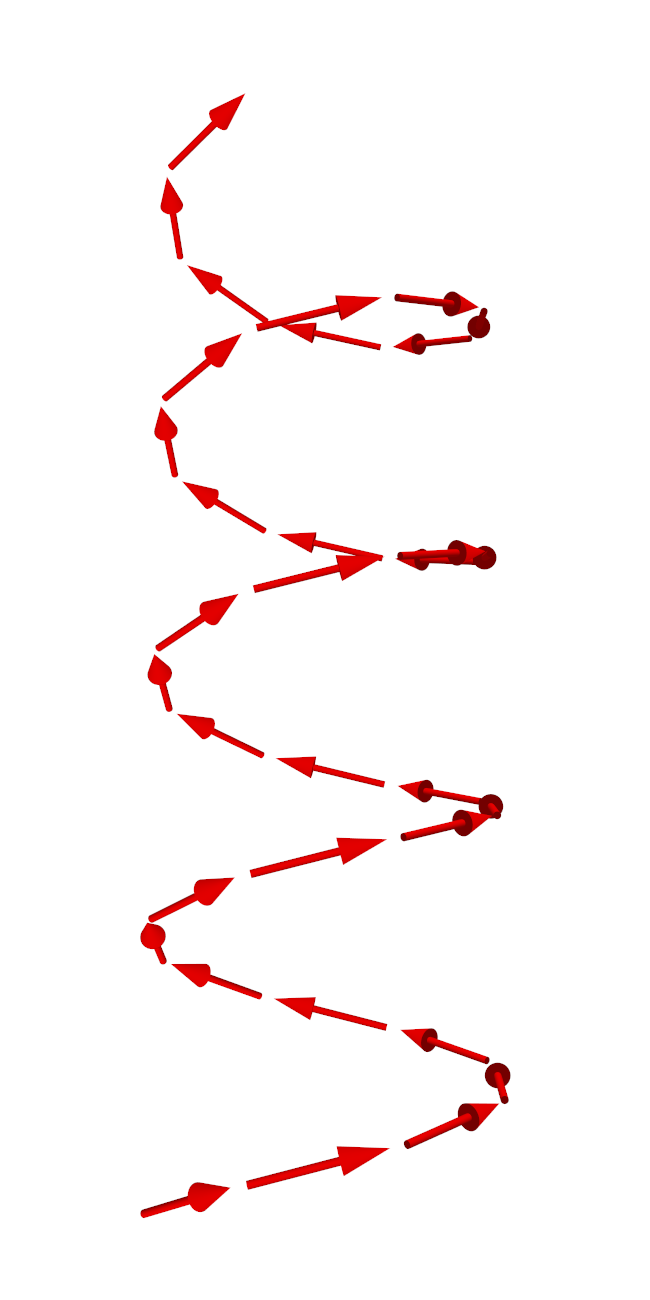﻿ VectorField – Algosim documentation
Algosim documentation: VectorField

# VectorField

Creates a vector field plot in ℝ² or ℝ³.

## Syntax

• `VectorField(f, ❨xmin, xmax[, δx]❩, ❨ymin, ymax[, δy]❩)`

• `f` is a function D → ℝ² where D ⊂ ℝ²

• `xmin`, `xmax`, `ymin`, and `ymax` are real numbers

• `δx` and `δy` are non-negative real numbers

• `VectorField(f, ❨xmin, xmax[, δx]❩, ❨ymin, ymax[, δy]❩, ❨zmin, zmax[, δz]❩)`

• `f` is a function D → ℝ³ where D ⊂ ℝ³

• `xmin`, `xmax`, `ymin`, `ymax`, `zmin`, and `zmax` are real numbers

• `δx`, `δy`, and `δz` are non-negative real numbers

• `VectorField(f, ❨xmin, xmax[, δx]❩, ❨ymin, ymax[, δy]❩, ❨zmin, zmax[, δz]❩)`

• `f` is a function D → ℝ⁴ where D ⊂ ℝ³

• `xmin`, `xmax`, `ymin`, `ymax`, `zmin`, and `zmax` are real numbers

• `δx`, `δy`, and `δz` are non-negative real numbers

• `VectorField(data)`

• `data` is a sequence of points in ℝ⁶ or ℝ⁷

## Description

### Planar vector fields

The `VectorField` function creates a plot of the vector field `f` in the rectangular region `[xmin, xmax] × [ymin, ymax]` with a sampling distance of `δx` in the first dimension and `δy` in the second dimension. The sampling distance is the distance between arrows. If omitted, a reasonable default value is used (typically producing a plot with about 20 arrows along each direction).

The plot is shown in the current diagram and a reference to the plot is returned.

### Vector fields in space

The `VectorField` function creates a plot of the vector field `f` in the box `[xmin, xmax] × [ymin, ymax] × [zmin, zmax]` with a sampling distance of `δx` in the first dimension, `δy` in the second, and `δz` in the third. The sampling distance is the distance between arrows. If omitted, a reasonable default value is used (typically producing a plot with about 20 arrows along each direction).

In addition to an ordinary vector field D → ℝ³, the `VectorField` function can also be given a mapping D → ℝ⁴ where each image is of the form `❨x, y, z, colour❩`, the fourth component being the integral representation of the arrow’s colour at `❨x, y, z❩`.

The plot is shown in the current scene and a reference to the plot is returned.

### Vector fields on parameterised manifolds

The above approach to plotting a vector field in space requires the domain to be a rectangular cuboid. The `VectorField(data)` approach relaxes this requirement; in particular, it lets the user plot a vector field along a curve or surface.

If `data` is a sequence of points in ℝ⁶ of the form `❨x, y, z, u, v, w❩`, then `VectorField(data)` draws the vector field obtained by plotting `❨u, v, w❩` at `❨x, y, z❩`. If `data` is a sequence of points in ℝ⁷ of the form `❨x, y, z, u, v, w, c❩`, where `c` is an integral colour code, the vector at `❨x, y, z❩` will be drawn in the colour `c`.

`data` may be a sequence of vectors, a single list of vectors, or an n×6 (n×7) matrix in which each row represents a single vector field arrow.

## Examples

```D ≔ diagram("vector field");
sf ≔ heatmap((x, y) ↦ x^2 + y^2, ❨−10, 10❩, ❨−10, 10❩, '("white", "red"));
vf ≔ VectorField((x, y) ↦ ❨2⋅x, 2⋅y❩, ❨−10, 10❩, ❨−10, 10❩)
``````D ≔ diagram("field");
disk(❨0, 0❩, .3);
F ≔ (x, y) ↦ ❨−y, x❩ / (x^2 + y^2)^1.5;
vf ≔ VectorField(F, ❨−2, 2, .5❩, ❨−2, 2, .5❩);
``````scene("field");
C ≔ cylinder(❨0, 0, −10❩, ❨1, 1, 20❩);
F ≔ (x, y, z) ↦ ❨−y, x, 0❩ / (x^2 + y^2)^.5;
VF ≔ VectorField(F, ❨−5, 5, 2❩, ❨−5, 5, 2❩, ❨−10, 10, 2❩)
``````NF ≔ F ↦ ((u, v) ↦ normalized(diff(F(u, v), u, u) × diff(F(u, v), v, v)));

S ≔ ClearScene("Möbius strip");

F ≔ (u, v) ↦ ❨(1 + .5⋅v⋅cos(u/2))⋅cos(u), (1 + .5⋅v⋅cos(u/2))⋅sin(u), .5⋅v⋅sin(u/2)❩;
M ≔ surf([0, 2⋅π] × [−1, 1] @ F);
"show parameter curves": true,
"parameter curve counts": ❨64, 16❩,
"line width": .75,
"unisided": true);

N ≔ NF(F);
VF ≔ VectorField([0, 2⋅π, π/32] × [−1, 1, 1/8] @ ((u, v) ↦ F(u, v) ~ N(u, v)));
"size": 0.1,
"anchor point": 1.03,
"color": "gold")
``````VF ≔ VectorField([−4⋅π, 4⋅π, π/4] @ (t ↦ ❨cos(t), sin(t), t/4, −sin(t), cos(t), 1/4❩));